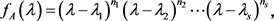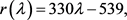# 矩阵的最小多项式及其应用Minimum Polynomial of Matrix and Its Application

DOI: 10.12677/AAM.2018.711159, PDF, HTML, XML, 下载: 602  浏览: 2,341

Abstract: Based on the theory of minimum polynomials, this paper introduces the method of finding the minimum polynomials with characteristic polynomials, and sums up the application of minimum polynomials in simplifying arbitrary matrix polynomials, finding dimensions and bases of linear spaces, solving matrix equations, and diagonalizing judgment matrices.

1. 前言

2. 利用特征多项式求最小多项式${m}_{A}\left(\lambda \right)={\left(\lambda -{\lambda }_{1}\right)}^{{d}_{1}}{\left(\lambda -{\lambda }_{2}\right)}^{{d}_{2}}\cdots {\left(\lambda -{\lambda }_{s}\right)}^{{d}_{s}},$ 其中 ${d}_{i}\le {n}_{i}\left(i=1,2,\dots ,s\right).$

${f}_{A}\left(\lambda \right)=|\lambda E-A|=\left(\lambda -1\right){\left(\lambda -2\right)}^{2},$

${m}_{1}\left(\lambda \right)=\left(\lambda -1\right)\left(\lambda -2\right)$

${m}_{1}\left(A\right)=\left(A-E\right)\left(A-2E\right)=O$

${m}_{A}\left(\lambda \right)=\left(\lambda -1\right)\left(\lambda -2\right).$

3. 最小多项式的应用

3.1. 化简任意矩阵多项式

${f}_{A}\left(\lambda \right)={\left(\lambda -2\right)}^{3},$

${m}_{1}\left(\lambda \right)=\left(\lambda -2\right)$${m}_{2}\left(\lambda \right)={\left(\lambda -2\right)}^{2}$${m}_{3}\left(\lambda \right)={\left(\lambda -2\right)}^{3}$

${m}_{1}\left(A\right)=\left(A-2E\right)\ne O$${m}_{2}\left(A\right)={\left(A-2E\right)}^{2}=O$

${m}_{A}\left(\lambda \right)={\left(\lambda -2\right)}^{2}$

$f\left(\lambda \right)=q\left(\lambda \right){m}_{A}\left(\lambda \right)+r\left(\lambda \right)$$f\left(A\right)=r\left(A\right)=330A-539E=\left[\begin{array}{ccc}121& 0& 0\\ 330& -209& 330\\ 330& -330& 451\end{array}\right]$

3.2. 求线性空间的维数和一组基

1) $W$ 的维数等于 ${m}_{A}\left(\lambda \right)$ 的次数 $k$ ，即 $\mathrm{dim}\left(W\right)=\partial \left({m}_{A}\left(\lambda \right)\right)=k$

2) $E,A,{A}^{2},\cdots ,{A}^{k-1}$$W$ 的一组。

${f}_{A}\left(\lambda \right)=\left(\lambda -1\right)\left(\lambda -w\right)\left(\lambda -{w}^{2}\right)$

${w}^{2}=\frac{-1-\sqrt{3}i}{2}$${w}^{3}=1$

$f\left(\lambda \right)$ 有三个互异的特征值，矩阵  的最小多项式为

${m}_{A}\left(\lambda \right)={f}_{A}\left(\lambda \right)=\left(\lambda -1\right)\left(\lambda -w\right)\left(\lambda -{w}^{2}\right)$

$\mathrm{dim}\left(W\right)=\partial \left({m}_{A}\left(\lambda \right)\right)=3$

3.3. 求解矩阵方程

$\stackrel{˜}{A}X=AX$$\underset{˜}{B}X=BX$

$\left({m}_{A}\left(\lambda \right),{m}_{B}\left(\lambda \right)\right)=1$

$X={\left(\stackrel{˜}{A}-\underset{˜}{B}\right)}^{-1}C=-\underset{k=0}{\overset{r-1}{\sum }}\underset{s=0}{\overset{k}{\sum }}{\alpha }_{r-s}{A}^{r-1-k}C{B}^{k-s}u\left(B\right)$

${m}_{A}\left(\lambda \right)u\left(\lambda \right)+{m}_{B}\left(\lambda \right)v\left(\lambda \right)=1$

$A=\left[\begin{array}{ccc}1& 1& 0\\ -1& -1& 1\\ -2& -2& 0\end{array}\right]$$B=\left[\begin{array}{ccc}0& 1& 0\\ 0& 0& 1\\ -1& -2& -1\end{array}\right]$$C=\left[\begin{array}{ccc}1& 0& 1\\ 0& 0& 0\\ 1& 0& 1\end{array}\right]$

${m}_{A}\left(\lambda \right)={\lambda }^{3}+2\lambda$${m}_{B}\left(\lambda \right)={\lambda }^{3}+{\lambda }^{2}+2\lambda +1$

${m}_{A}\left(\lambda \right)\left(-{\lambda }^{2}-\lambda -1\right)+{m}_{B}\left(\lambda \right)\left({\lambda }^{2}+1\right)=1$

$u\left(\lambda \right)=-\left({\lambda }^{2}+\lambda +1\right)$ ，从而

$u\left(B\right)=-\left({B}^{2}+B+1\right)=\left[\begin{array}{ccc}-1& -1& -1\\ 1& 1& 0\\ 0& 1& 1\end{array}\right]$

${\alpha }_{1}=2,{\alpha }_{2}=0,{\alpha }_{3}=1$ ，代入

$X={\left(\stackrel{˜}{A}-\underset{˜}{B}\right)}^{-1}C=-\underset{k=0}{\overset{r-1}{\sum }}\underset{s=0}{\overset{k}{\sum }}{\alpha }_{r-s}{A}^{r-1-k}C{B}^{k-s}u\left(B\right)$

$X=-\left(-{A}^{2}C+ACB+C{B}^{2}+2C\right)u\left(B\right)=\left[\begin{array}{ccc}3& 1& 1\\ -3& 0& 0\\ 0& -2& 1\end{array}\right]$

3.4. 在矩阵对角化中的应用

1)  ；2) ${A}^{2}=A$

2) ${A}^{2}=A$$f\left(\lambda \right)={\lambda }^{2}-\lambda =\lambda \left(\lambda -1\right)$$A$ 的零化多项式，因为特征方程无重根，所以 $A$ 能够对角化。

4. 结论

  龙小胖. 最小多项式的求法[J]. 井冈山大学学报(社会科学版), 2004, 25(5): 54-55.  夏必腊. 方阵最小多项式的性质与求法[J]. 高等数学研究, 2003, 6(3): 34-39.  陕振沛, 姚景景, 陈华平. 基于矩阵特征多项式与最小多项式相关的探讨[J]. 六盘水师范院学报, 2013, 25(5): 55-58.  胡瑞平. 矩阵方程的最小多项式解法[J]. 应用数学学报, 1993, 16(3): 295-301.  王莲花, 王建平, 李艳华. 最小多项式的性质及其应用[J]. 河南教育学院学报(自然科学版), 2004, 13(2): 12-13.  靳艳芳. 最小多项式的性质、求法和应用[J]. 综合学术论坛, 2010, 17(5): 209-212.